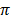# Profit Maximization Model of a Firm (With Diagram)

The efficient management of a business firm requires an optimal or best solution out of the available courses of action for a firm.

This efficient or optimal decision making requires establishing the goal or objective to be achieved.

Whether a management decision is optimal or not can be evaluated against the goal or objective that the firm seeks to achieve.

#### 1. Profit Maximisation Model:

In traditional economic model of the firm it is assumed that a firm’s objective is to maximise short-run profits, that is, profits in the current period which is generally taken to be a year. In various forms of market structure such as perfect competition, monopoly, monopolistic competition the traditional microeconomic theory explains the determination of price and output by assuming that firm’s aim is to maximise current or short-run profits. This current short-run profit maximisation model of the firm has provided decision makers with useful framework with regard to efficient management and allocation of resources.

Profit is a difference between total revenue and total cost. It may be noted that the concept of cost used in economic theory and managerial economics is different from the concept of accounting cost used by accountants. This difference in the concepts of costs makes the concept of profits used in economic theory different from that used in its calculation by the accountant.

It is to state here that economic profits is the difference between total revenue and economic costs. Thus,

Wherestands for total economic profits, TR for total revenue and TC for total economic costs. It is economic profits which firms try to maximise in their decision making about level of output to be produced and price to be charged for its product. This is illustrated in Fig.2.1. where TR curve represents total revenue earned from selling varying amounts of output of a product. TC curve depicts total economic costs at different levels of output. It will be seen from the upper part of Fig.2.1 at OM level of output, total revenue equals total economic costs and therefore at this level of output the firm is just breaking even.

Therefore, point B at which TR curve cuts TC curve is called break-even point. Beyond this break-even level of output positive profits start accruing to the firm as it expands its level of output. Profits go on increasing till output level OQ is reached. It will be seen from the upper part of Figure 2.1 that at output OQ, the difference between total revenue and total cost is maximum, that is, JH is the largest distance between the TR and TC curves.

Therefore, JH is the maximum profits that can be earned by the firm, given the total revenue and total cost conditions.

That the profits are maximum at output level OQ can be shown mathematically as under:

For the total profits to be maximum, the first derivative of the total profit function should be zero. Thus, taking the first derivative of (1) above we have

or , d (TR)/ dQ= d(TC) dQ

d (TR) /dQ and d(TC) dQ and are the slopes of TR and TC curves respectively.

It will be seen from Fig.2.1 that the slope of the TR and TC curves corresponding to output level OQ are the same as the slope of the tangents drawn to these curves are the same at this output level.

In the lower part of Figure 2.1 we have drawn a total profit curve TP which first rises and then beyond point N (corresponding to output level OQ) it starts falling indicating that profits are maximum at output level OQ.

It can be seen from the upper part of Figure 2.1 that profits start declining as output is expanded beyond OQ. Therefore, a firm which aims to maximise profits will produce output level of OQ, and will charge a price of its product which buyers are prepared to pay depending on the demand conditions.

Since price or average revenue equals total revenue divided by a level of output, price charged by the firm at output level OQ is given TR/OQ or QJ/OQ

The simple profit-maximizing model of the firm provides very useful guidelines for the decision making by the firm with regard to efficient resource management.

Thus, any business decision by a firm will increase its profits if the following conditions prevail:

1. It brings about increase in total revenue more than increase in costs.

2. It causes increase in revenue, costs remaining unchanged.

3. It reduces cost more than it reduces revenue.